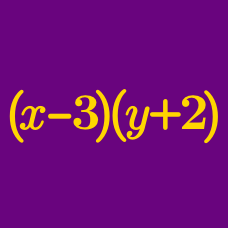Algebra

# Multiplying and Dividing Monomials

Simplify $\frac{1}{2} ab \times \left(-a^2b\right).$

Simplify the following expression:

$16 x^3 \div \left( \frac{4}{3} x^2 \right).$

Simplify $a^{-2} b^3 \times a^5 b^{-2} \div \left( a^2 b \right).$

A rectangular cuboid with its base measuring $$4ab^2 \text{ cm}$$ by $$3a^2 \text{ cm}$$ has a volume of $$36a^5b^6 \text{ cm}^3$$. What is its height in $$\text{cm}$$?

Simplify $\frac{9 a^2 b \times 2 c}{36 a c^2} .$

×